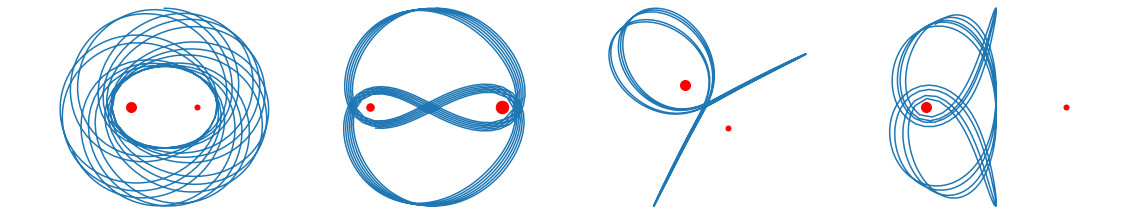# Mathematical PythonMathematical Python is an introduction to mathematical computing including:

• Jupyter notebooks, markdown and $\LaTeX$
• Basic Python programming: datatypes, variables, logic, loops and functions
• Scientific computing with NumPy, SciPy and Matplotlib
• Applications in calculus, linear algebra and differential equations

## Prerequisites

• Differential calculus: derivatives, Taylor series and optimization
• Integral calculus: integrals, Riemann sums, sequences and series
• Linear algebra: vector and matrix operations, systems of equations, eigenvalues and eigenvectors
• Differential equations: first and second order equations, Euler's method and systems of equations

## Author

Patrick Walls is Associate Professor of Teaching in the Department of Mathematics at the University of British Columbia.

## Feedback

August 16 2022 14:12 PST